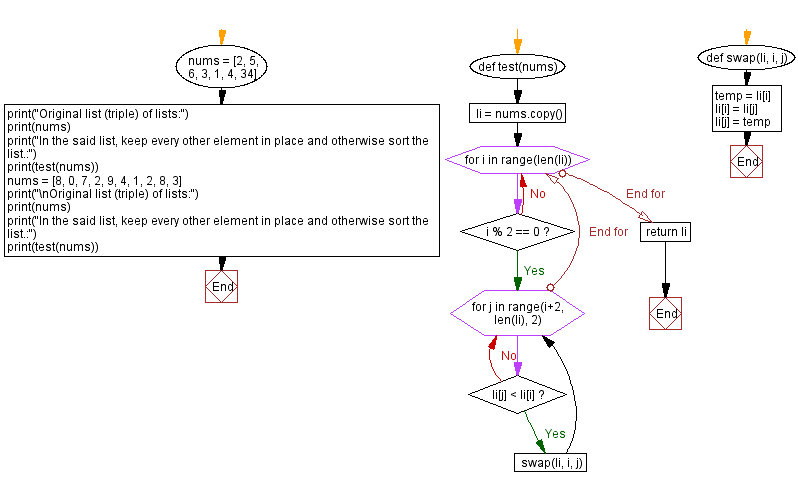﻿ Python: Start with a list of integers, keep every other element in place and otherwise sort the list - w3resource# Python: Start with a list of integers, keep every other element in place and otherwise sort the list

## Python Programming Puzzles: Exercise-92 with Solution

Write a Python program to start with a list of integers, keep every other element in place and otherwise sort the list.

```Input:
[2, 5, 6, 3, 1, 4, 34]
Output:
[1, 5, 2, 3, 6, 4, 34]

Input:
[8, 0, 7, 2, 9, 4, 1, 2, 8, 3]
Output:
[1, 0, 7, 2, 8, 4, 8, 2, 9, 3]
```

Sample Solution:

Python Code:

``````#License: https://bit.ly/3oLErEI

def test(nums):
li = nums.copy()
for i in range(len(li)):
if i % 2 == 0:
for j in range(i+2, len(li), 2):
if li[j] < li[i]:
swap(li, i, j)

return li
def swap(li, i, j):
temp = li[i]
li[i] = li[j]
li[j] = temp
nums = [2, 5, 6, 3, 1, 4, 34]
print("Original list (triple) of lists:")
print(nums)
print("In the said list, keep every other element in place and otherwise sort the list.:")
print(test(nums))
nums = [8, 0, 7, 2, 9, 4, 1, 2, 8, 3]
print("\nOriginal list (triple) of lists:")
print(nums)
print("In the said list, keep every other element in place and otherwise sort the list.:")
print(test(nums))
``````

Sample Output:

```Original list (triple) of lists:
[2, 5, 6, 3, 1, 4, 34]
In the said list, keep every other element in place and otherwise sort the list.:
[1, 5, 2, 3, 6, 4, 34]

Original list (triple) of lists:
[8, 0, 7, 2, 9, 4, 1, 2, 8, 3]
In the said list, keep every other element in place and otherwise sort the list.:
[1, 0, 7, 2, 8, 4, 8, 2, 9, 3]
```

Flowchart:## Visualize Python code execution:

The following tool visualize what the computer is doing step-by-step as it executes the said program:

Python Code Editor :

Have another way to solve this solution? Contribute your code (and comments) through Disqus.

What is the difficulty level of this exercise?

Test your Programming skills with w3resource's quiz.

﻿

## Python: Tips of the Day

Clamps num within the inclusive range specified by the boundary values x and y:

Example:

```def tips_clamp_num(num,x,y):
return max(min(num, max(x, y)), min(x, y))
print(tips_clamp_num(2, 4, 6))
print(tips_clamp_num(1, -1, -6))
```

Output:

```4
-1
```